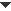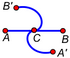﻿ Objects > Object Categories > Path Objects

# Path Objects

Navigation:  Objects > Object Categories >

# Path ObjectsThere are certain geometric objects in Sketchpad on which you can construct and animate points. These objects are collectively referred to as path objects. Path objects include:

straight objects (segments, rays, lines, and axes)

arcs

polygons and other interiors (the perimeter of an interior forms that interior’s path)

point loci, function plots, parametric plots, and sampled transformed paths

There are several commands that apply to path objects:

Use Construct | Point on Object to construct a point on a path.

Use Display | Line Style to set the style of most paths.

Use Measure | Value of Point to measure the value of a point on a path.

Use Graph | Plot Value on Path to plot a point at a particular position on a path.Animate a Point on a Path
 When a point is constructed on a path, you can animate the point along the path, either by choosing Display | Animate or by creating an Animation button. To animate an independent point along a path, you must first merge the point to the path.Construct a Locus Using a Path as the Domain
 If the intended locus driver is a point on a path, the path is automatically used as the domain for the driver. Select the driver and the driven object. Then choose If the intended locus driver is an independent point, you must select the path to use it as the domain. Select the driver, the path, and the driven object. Then chooseAuto-Dashing of Coincident or Collinear Paths
 When you construct a path coinciding with, or collinear to, a longer path (for example, when you construct an arc on the circumference of a circle, or when you construct a segment along a line), the longer path becomes dashed to better display the shorter path coinciding with it. Since a dashed coordinate axis is seldom desirable, a straight object constructed on an axis is drawn one step thicker than the default line thickness.Transform a Path Using Translation, Rotation, Dilation, and Reflection
 Use Sketchpad’s built-in transformations to create a transformed image of a path. Select the picture and choose or Dilate from the Transform menu. Depending on the transformation you want, you may need to mark various objects or values to serve as center, distance, angle, ratio, or mirror. These four transformations, and any transformation that combines them, are called similarity transformations because the transformed image is similar to the original: angles and ratios of distances are preserved.Transform a Path Using a Custom Transformation
 Use Sketchpad’s custom transformations to create an arbitrary transformed image of a path. Use two points, one of which depends on the other, to define a custom transformation. Select the path you want to transform. Choose the custom transformation from the bottom of the Transform menu. If the two points that define the custom transformation are related using only Sketchpad’s built-in transformations, the result is a normal transformed path, just as if you had applied the various transformations directly to the original path. If the pre-image path is a segment, the image remains a segment; if the pre-image is a circle, so is the image. If the two points that define the custom transformation are related in some other way, the result is a sampled transformed path.Sampled Transformed Paths
 A sampled transformed path is a custom transformed image of a path object in which the custom transformation doesn’t rely strictly on Sketchpad’s built-in transformations of translation, rotation, reflection, and dilation. Transformed images constructed using the built-in transformations retain their essential shapes as straight objects, circles, arcs, polygons, and so forth. Transformed images constructed in other ways may not retain the shapes of their pre-images. For instance, horizontal scaling transforms a circle into an ellipse, and swirling transforms a segment into a curve.Such transformed images must be displayed using a sampling process: The transformed path is displayed by sampling locations along the pre-image and then transforming each sample to create the transformed image. The smoothness and level of detail of the transformed path depend on the number of samples used. To change the number of samples and whether the transformed path is displayed as continuous or discrete, select the transformed path and choose

When polygons and other interiors are transformed in this way, only the border of the interior is transformed.